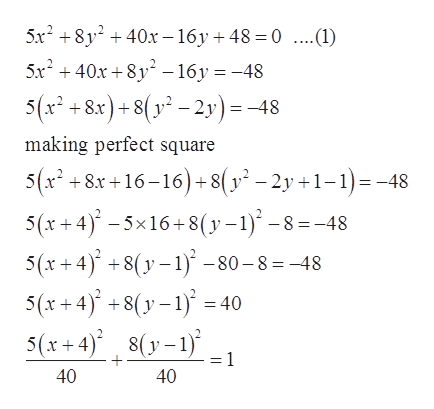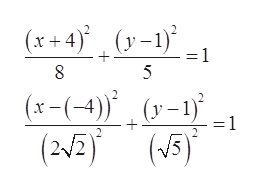# For Exercise,a. Write the equation of the ellipse in standard form.b. Identify the center, vertices, endpoints of the minor axis, and foci.5x2 + 8y2 + 40x − 16y + 48 = 0

Question
4 views

For Exercise,

a. Write the equation of the ellipse in standard form.

b. Identify the center, vertices, endpoints of the minor axis, and foci.

5x2 + 8y2 + 40x − 16y + 48 = 0

check_circle

Step 1

Given:

Step 2

Standard form of ellipse ishelp_outlineImage Transcriptionclose5x? + 8y? + 40x – 16y + 48 = 0 ..(1) 5x² + 40x + 8y – 16y = -48 5(x² + 8x) + 8( y² – 2y)=-48 making perfect square + 8x +16–16)+8(y² – 2y +1–1)=-48 5(x + 4)' – 5×16 +8(y – 1)´ – 8 = -48 5(x +4) +8(y – 1)° – 80 – 8=-48 5(x + 4)* +8(y – 1) = 40 5(x + 4)° 8(y–1) = 1 40 40 fullscreen
Step 3

Further calcul...help_outlineImage Transcriptionclose(x + 4)° (y-1)° . :1 5 (x-(-4)" , (v–1) (2V2) (5) = 1 fullscreen

### Want to see the full answer?

See Solution

#### Want to see this answer and more?

Solutions are written by subject experts who are available 24/7. Questions are typically answered within 1 hour.*

See Solution
*Response times may vary by subject and question.
Tagged in
MathAlgebra

### Other